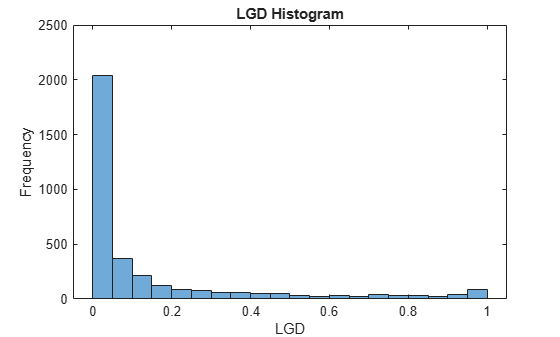# Compare Loss Given Default Models Using Cross-Validation

This example shows how to compare loss given default (LGD) models using cross-validation.

Load the LGD data. This data set is simulated for illustration purposes.

```load LGDData.mat disp(head(data))```
``` LTV Age Type LGD _______ _______ ___________ _________ 0.89101 0.39716 residential 0.032659 0.70176 2.0939 residential 0.43564 0.72078 2.7948 residential 0.0064766 0.37013 1.237 residential 0.007947 0.36492 2.5818 residential 0 0.796 1.5957 residential 0.14572 0.60203 1.1599 residential 0.025688 0.92005 0.50253 investment 0.063182 ```

The histogram of LGD values for this data set shows a significant number of values at or near `0` (full recovery) and only a relatively small fraction of values at or near `1` (total loss).

```histogram(data.LGD) xlabel('LGD') ylabel('Frequency') title('LGD Histogram')```### Cross-Validate Models

Compare three `Tobit` LGD models by varying the censoring side choice between the three supported options (`"both"`, `"left"`, and `"right"`). For more information, see the `'CensoringSide'` name-value argument for a `Tobit` object.

Use the `cvpartition` function to generate random partitions on the data for a k-fold cross-validation. For each partition, fit a `Tobit` model on the training data with each of the censoring side options and then obtain two validation metrics using the test data. This example uses the validation metrics for area under the receiver operating characteristic curve (AUROC) and the R-squared metric. For more information, see `modelDiscrimination` and `modelCalibration`.

```NumFolds = 10; rng('default'); % For reproducibility c = cvpartition(height(data),'KFold',NumFolds); ModelCensoringSide = ["both" "left" "right"]; NumModels = length(ModelCensoringSide); AUROC = zeros(NumFolds,NumModels); RSquared = zeros(NumFolds,NumModels); for ii=1:NumFolds fprintf('Fitting models, fold %d\n',ii); % Get the partition indices. TrainInd = training(c,ii); TestInd = test(c,ii); % For each model, fit with training data, measure with test data. for jj=1:NumModels % Fit the model with training data. lgdModel = fitLGDModel(data(TrainInd,:),'Tobit','CensoringSide',ModelCensoringSide(jj)); % Measure the model discrimination on test data. DiscMeasure = modelDiscrimination(lgdModel,data(TestInd,:)); AUROC(ii,jj) = DiscMeasure.AUROC; % Measure the model calibration on test data. CalMeasure = modelCalibration(lgdModel,data(TestInd,:)); RSquared(ii,jj) = CalMeasure.RSquared; end end```
```Fitting models, fold 1 Fitting models, fold 2 Fitting models, fold 3 Fitting models, fold 4 Fitting models, fold 5 Fitting models, fold 6 Fitting models, fold 7 Fitting models, fold 8 Fitting models, fold 9 Fitting models, fold 10 ```

Visualize the results for a selected metric for the three models side-by-side.

```SelectedMetric ="R-squared"; if SelectedMetric=="AUROC" PlotData = AUROC; else PlotData = RSquared; end bar(1:NumFolds,PlotData) xlabel('Fold') ylabel(SelectedMetric) title('Validation Metric by Fold') legend(ModelCensoringSide,'Location','southeast') grid on```The AUROC values for the three models are comparable across the folds, indicating that the three versions of the model effectively separate the low LGD and high LGD cases.

Regarding accuracy, the R-squared metric is low for the three models. However, the "`right"` censored model shows a lower R-squared metric than the other two models across the folds. The observed LGD data has many observations at or near `0` (total recovery). To improve the accuracy of the models, include an explicit limit at `0` when censoring on the `"left"` and on `"both"` sides.